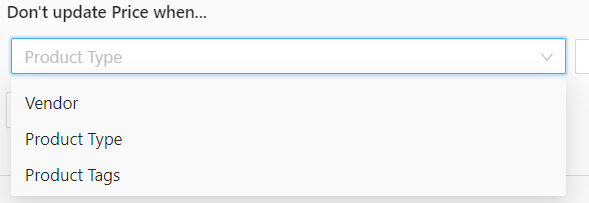Price Condition is available for all eCommerce platforms. Only certain conditions are not available for all.

Where is the Price Condition?

1. You will need to navigate to the specific Feed Settings > Field Mapping.
2. Price Condition is an extra setting under the Price field.

How to use Price Condition according to examples below:

The first tab is the statement and the second tab is the condition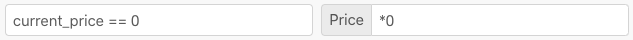`This condition will not update when the price in your store is 0 in the feed file`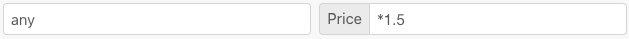`If the price is 11, then the price is + 1`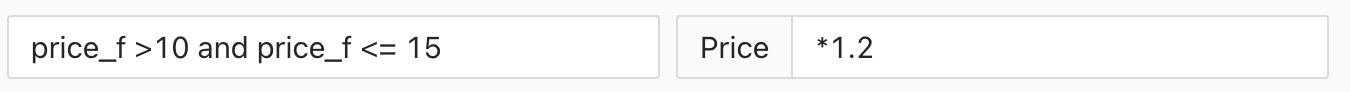`If the price is more than 10 and equals or below 15, then the price is multiplied by 1.2 or 20%`
`Use price_f when the comparison values are numeric``All the prices will be multiplied by 1.5 or 50%``If the price is more than column 1, then add column 1 and column 2 to the price`
`Use col#_f when the comparison values are numeric`

`Note: col expression is not available for the XML file format`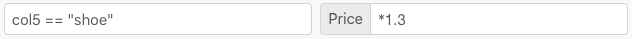`If column 5 has value shoe, then multiply by 1.3. This can be used for any column and is suitable if you are planning to add markup based on collections`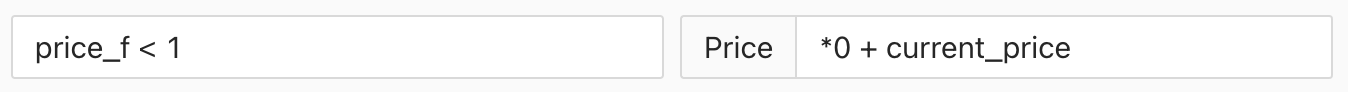`If the price is less than 1, then it will use the current price in store (Shopify only)`
`Use price_f when the comparison values are numeric``If the price is equal as "high", then it will multiply by 1.2`
`Use price when the comparison values are text`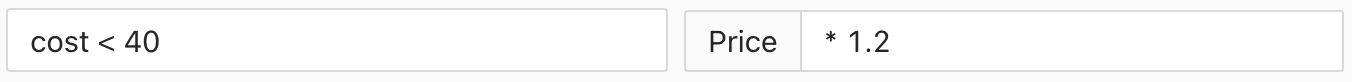`If cost in the store is less than 40, then multiply the price by 1.2. The cost value will be taken from the store. (Shopify only)``If compare at price in the store is more than 10, then multiply the price by 1.5. The compare at price value will be taken from the store. (Shopify only)`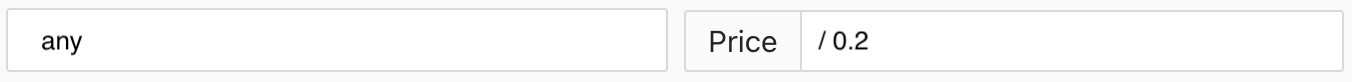`All the prices will be divided by 0.2````If the col4 date is more than current_timestamp, then multiply by 1.2

Use col4_date when the comparison values are date
now refers to current_timestamp```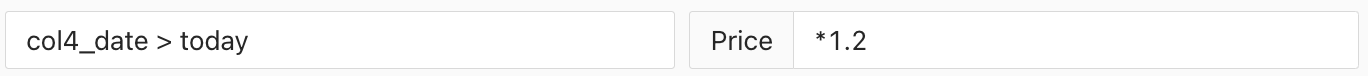```If the col4 date is more than today's date, then multiply by 1.2

Use col4_date when the comparison values are date
today refers to today's date```

Additional info on Price Condition

1. The Price Conditions take effect according to the arrangement. The first conditions have the highest priority.

2. Stock Sync now supports Column Value.

3. Valid price range keywords are:

• price (Use it when the comparison value are text)
• price_f (Use it when the comparison value are numeric)
• col#_f (Use it when the comparison value are numeric)
• any
• current_price (Shopify only)
• cost (Shopify only)
• compare_at_price (Shopify only)

4. The symbol that is used for the statement:

 Symbol Details == equal to <= less than or equal to >= more than or equal to > more than < less than and only true when both statements are true or true when either statement is true

5. There is another method for pricing conditions that you can use:-
For example, col13 = 'ABCD'Formulae Explanation contains('BC',col13) If you want to select only 'BC' from the whole text 'ABCD' contains('Large' , col13) != true Don't update the price when it contains the word Large!= (Not Equal) left(col13, 2) == 'AB' If you want to select only 2 letters from the left side of the whole text. right(col13, 2) == 'CD' If you want to select only 2 letters from the right side of the whole text. mid(col13, 2, 2) == 'BC' If you want to select only 2 letters in the middle of the whole text. len(col13) == 4 If you want to select the whole text, the total number of letters must be indicated there. Example: 4 indicated the length of the letters. find('BC', col13) == 2 If you want to find only 2 letters from the whole text. substitute(col13, 'BC', 'XY') == 'AXYD' If you want to substitute selected text from the whole text and replace it with another alphabet max(price, col2, col3) max(12,10,9) == 12If you want to take the maximum value from the three-column you can use this method. min(price, col2, col3) min(12,10,9) == 9If you want to take the minimum value from the three-column you can use this method.

6. Price Delimiter provided in price field which has two options one is Comma and Decimal Point.7.  Price Round is also available in the price field. You can choose any that is suitable for you. Click on the Price Round to know more.8. For the pricing condition, there is another way that can filter the price only according to the vendor, product type, and product tags. You can choose any one of the methods to filter the price.